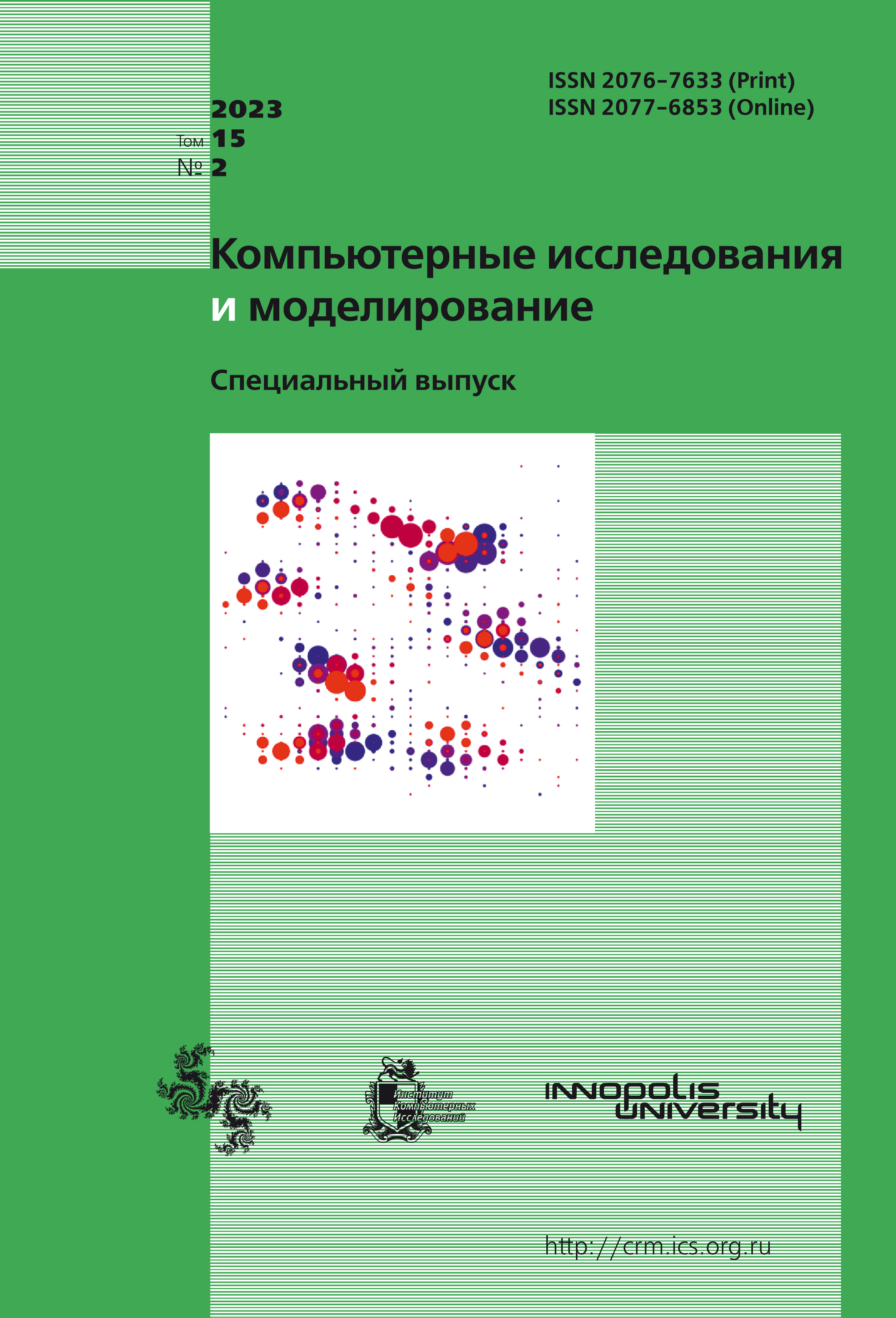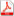Latest issue Issue 2, 2023 Vol. 15

# All issues

On the modification of the method of component descent for solving some inverse problems of mathematical physicspdf (861K)

The article is devoted to solving ill-posed problems of mathematical physics for elliptic and parabolic equations, such as the Cauchy problem for the Helmholtz equation and the retrospective Cauchy problem for the heat equation with constant coefficients. These problems are reduced to problems of convex optimization in Hilbert space. The gradients of the corresponding functionals are calculated approximately by solving two well-posed problems. A new method is proposed for solving the optimization problems under study, it is component-by-component descent in the basis of eigenfunctions of a self-adjoint operator associated with the problem. If it was possible to calculate the gradient exactly, this method would give an arbitrarily exact solution of the problem, depending on the number of considered elements of the basis. In real cases, the inaccuracy of calculations leads to a violation of monotonicity, which requires the use of restarts and limits the achievable quality. The paper presents the results of experiments confirming the effectiveness of the constructed method. It is determined that the new approach is superior to approaches based on the use of gradient optimization methods: it allows to achieve better quality of solution with significantly less computational resources. It is assumed that the constructed method can be generalized to other problems.

Keywords: inverse problems, convex optimization, optimization in a Hilbert space, first order methods, component descent, imprecise oracle
Citation in English: Pletnev N.V., Matyukhin V.V. On the modification of the method of component descent for solving some inverse problems of mathematical physics // Computer Research and Modeling, 2023, vol. 15, no. 2, pp. 301-316
Citation in English: Pletnev N.V., Matyukhin V.V. On the modification of the method of component descent for solving some inverse problems of mathematical physics // Computer Research and Modeling, 2023, vol. 15, no. 2, pp. 301-316
DOI: 10.20537/2076-7633-2023-15-2-301-316

Full-text version of the journal is also available on the web site of the scientific electronic library eLIBRARY.RU

The journal is included in the Russian Science Citation Index

The journal is included in the List of Russian peer-reviewed journals publishing the main research results of PhD and doctoral dissertations.

International Interdisciplinary Conference "Mathematics. Computing. Education"

The journal is included in the RSCI

Indexed in Scopus